### Linear Predictor, Predicted Probability, and Confidence Limits

This section describes how predicted probabilities and confidence limits are calculated by using the maximum likelihood estimates (MLEs) obtained from PROC LOGISTIC. For a specific example, see the section Getting Started: LOGISTIC Procedure. Predicted probabilities and confidence limits can be output to a data set with the OUTPUT statement.

#### Binary and Cumulative Response Models

For a vector of explanatory variables, the linear predictor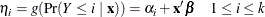is estimated by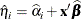where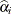and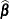are the MLEs of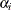and. The estimated standard error of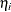is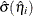, which can be computed as the square root of the quadratic form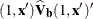, where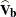is the estimated covariance matrix of the parameter estimates. The asymptotic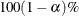confidence interval foris given by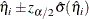where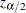is the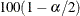percentile point of a standard normal distribution.

The predicted probability and theconfidence limits for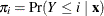are obtained by back-transforming the corresponding measures for the linear predictor, as shown in the following table:

Predicted Probability

100(1–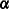)% Confidence Limits

LOGIT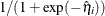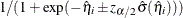PROBIT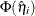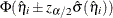CLOGLOG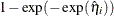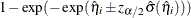The CONTRAST statement also enables you to estimate the exponentiated contrast,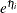. The corresponding standard error is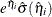, and the confidence limits are computed by exponentiating those for the linear predictor: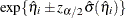.

#### Generalized Logit Model

For a vector of explanatory variables, define the linear predictors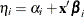, and let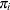denote the probability of obtaining the response value i: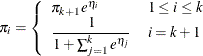By the delta method,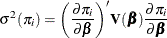A 100(1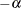)% confidence level foris given by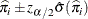where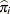is the estimated expected probability of response i, and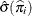is obtained by evaluating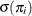at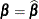.

Note that the contrast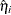and exponentiated contrast, their standard errors, and their confidence intervals are computed in the same fashion as for the cumulative response models, replacingwith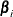.